Скачать презентацию Conflict-Free Coloring Gila Morgenstern CRI Haifa University

76ec41212693d4c73d020de5303f6ac0.ppt

• Количество слайдов: 35Conflict-Free Coloring Gila Morgenstern CRI, Haifa University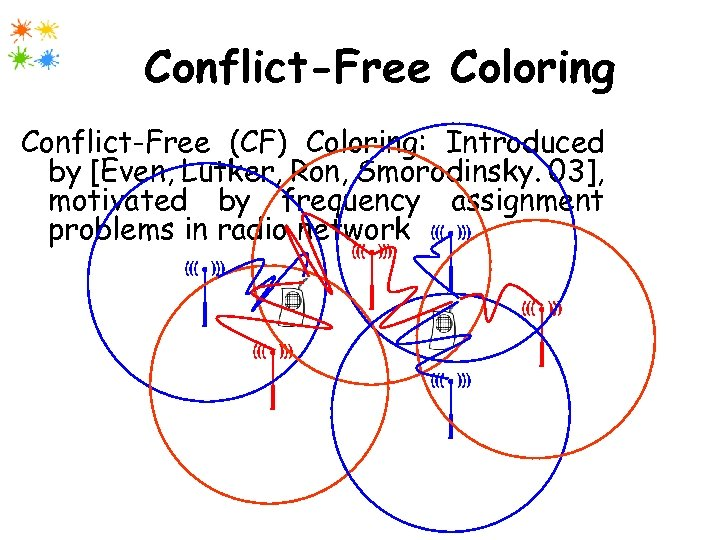Conflict-Free Coloring Conflict-Free (CF) Coloring: Introduced by [Even, Lutker, Ron, Smorodinsky. 03], motivated by frequency assignment problems in radio network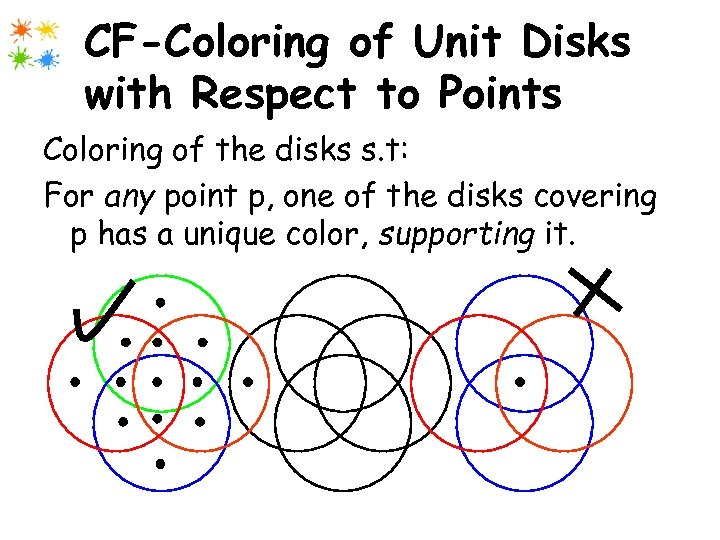CF-Coloring of Unit Disks with Respect to Points Coloring of the disks s. t: For any point p, one of the disks covering p has a unique color, supporting it.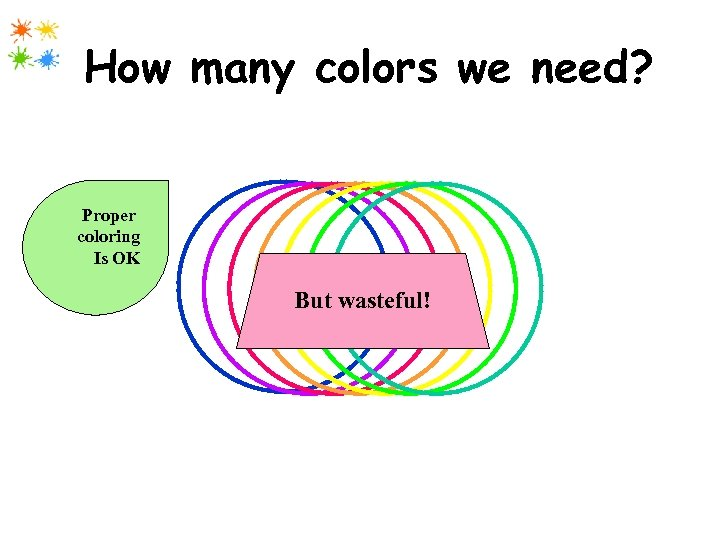How many colors we need? Proper coloring Is OK But wasteful!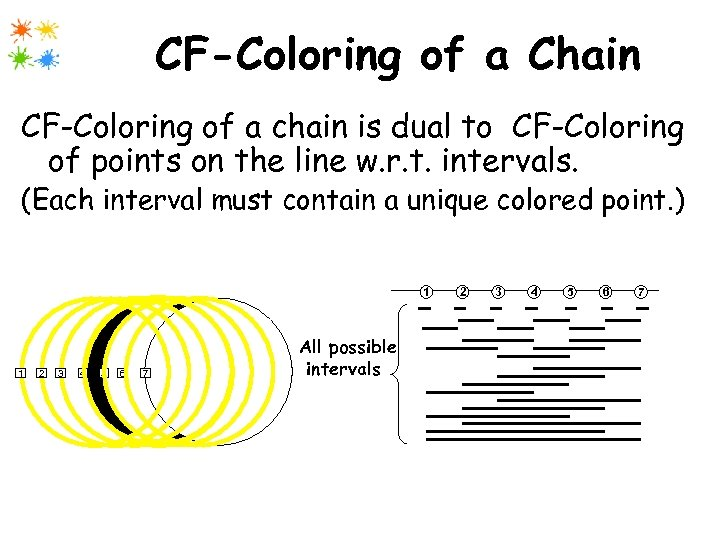CF-Coloring of a Chain CF-Coloring of a chain is dual to CF-Coloring of points on the line w. r. t. intervals. (Each interval must contain a unique colored point. ) 1 1 2 3 4 5 6 7 All possible intervals 2 3 4 5 6 7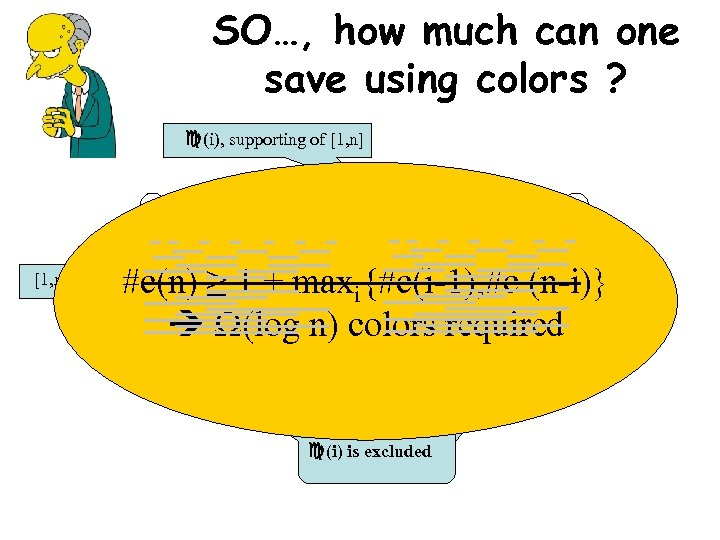SO…, how much can one save using colors ? (i), supporting of [1, n] 1 [1, n] 2 3 i n #c(n) ≥ 1 + maxi{#c(i-1), #c (n-i)} Ω(log n) colors required Independent, (i) is excluded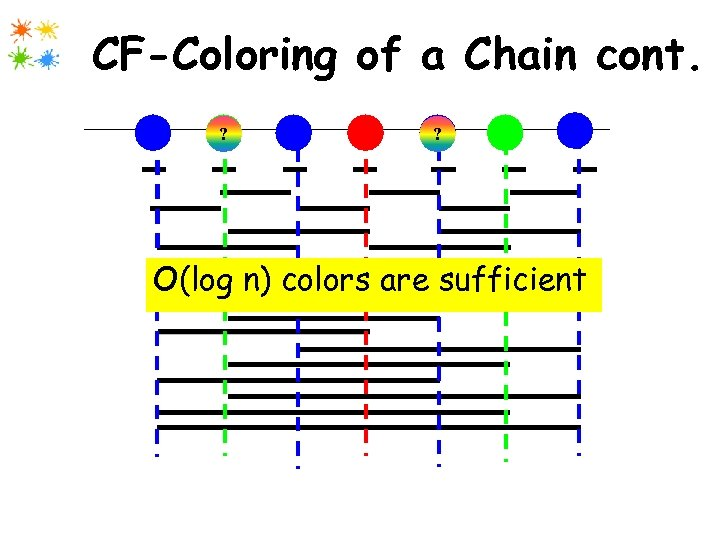CF-Coloring of a Chain cont. 1 2 ? 3 4 5 ? 6 7 O(log n) colors are sufficient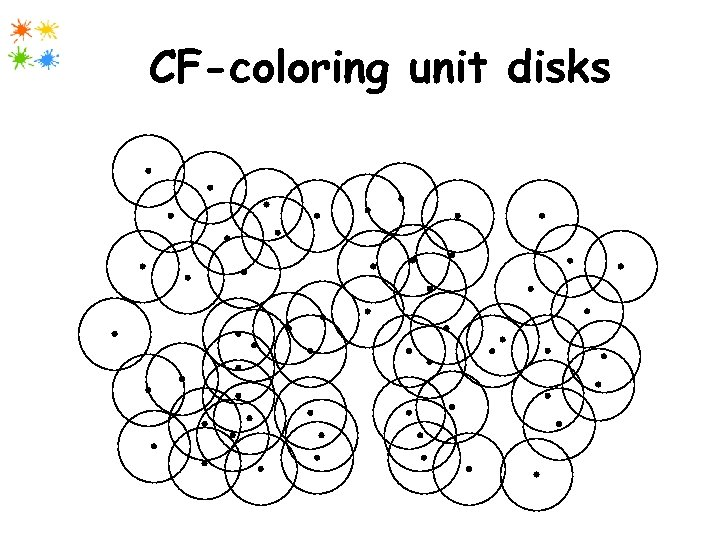CF-coloring unit disks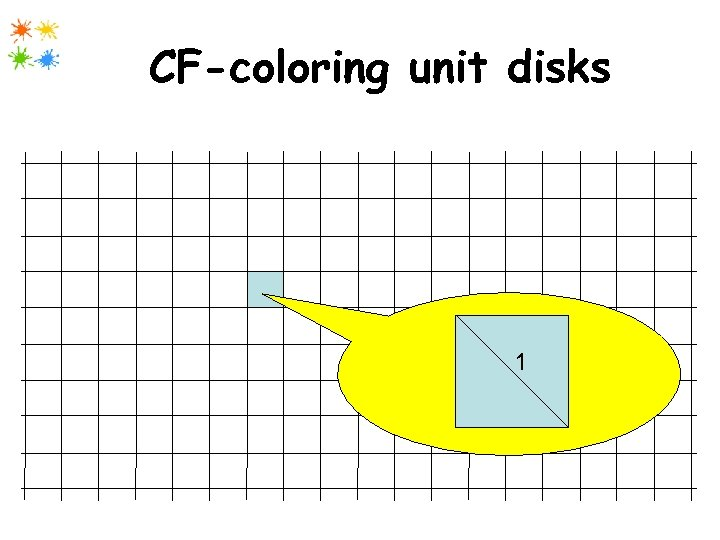CF-coloring unit disks 1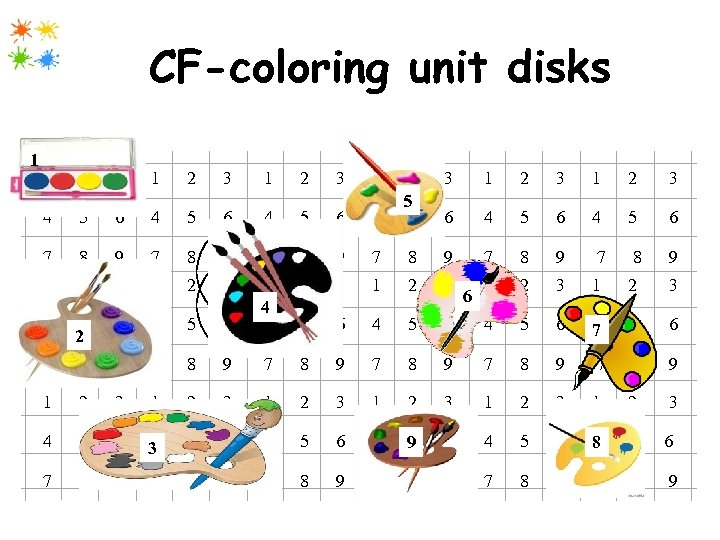CF-coloring unit disks 1 1 2 3 1 2 3 4 2 5 5 4 5 6 6 4 5 6 7 8 9 7 8 9 1 2 3 4 5 2 6 4 5 6 1 4 4 2 3 1 2 3 5 6 4 7 5 6 7 8 9 7 8 9 1 2 3 1 2 3 4 5 6 4 3 5 6 4 5 9 6 4 5 6 4 8 5 6 7 8 9 7 8 9 6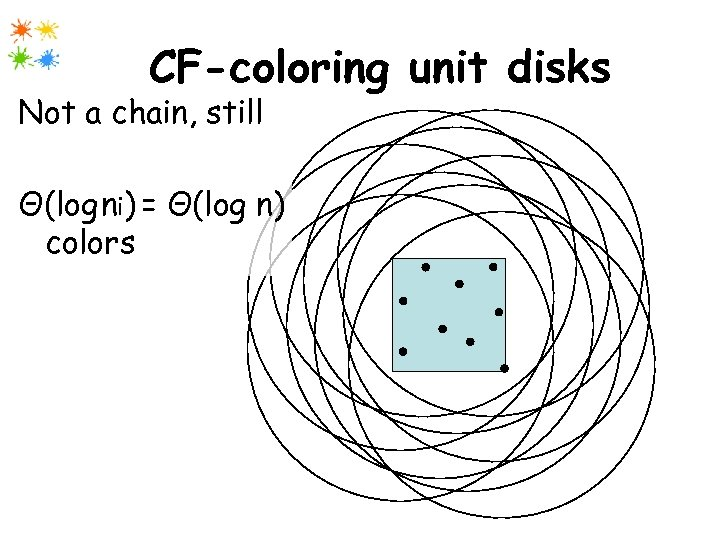CF-coloring unit disks Not a chain, still Θ(log ni) = Θ(log n) colors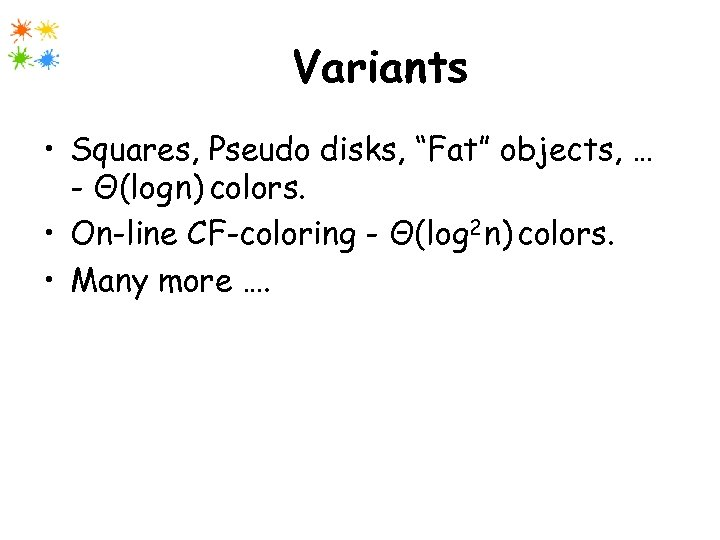Variants • Squares, Pseudo disks, “Fat” objects, … - Θ(log n) colors. • On-line CF-coloring - Θ(log 2 n) colors. • Many more ….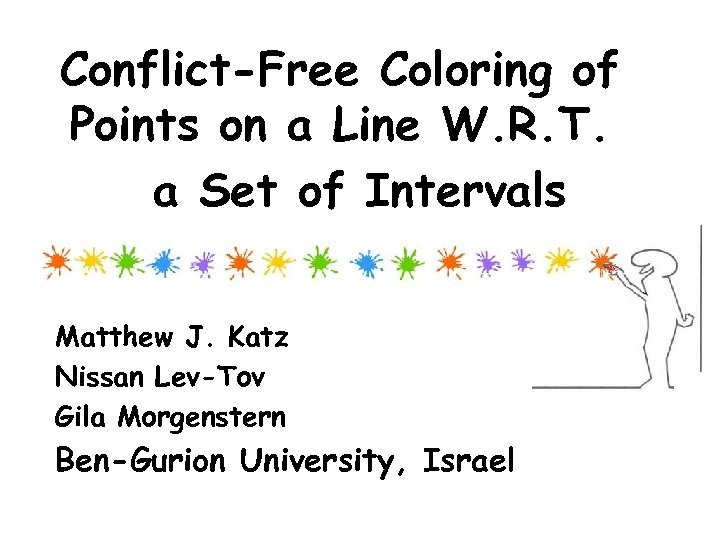Conflict-Free Coloring of Points on a Line W. R. T. a Set of Intervals Matthew J. Katz Nissan Lev-Tov Gila Morgenstern Ben-Gurion University, Israel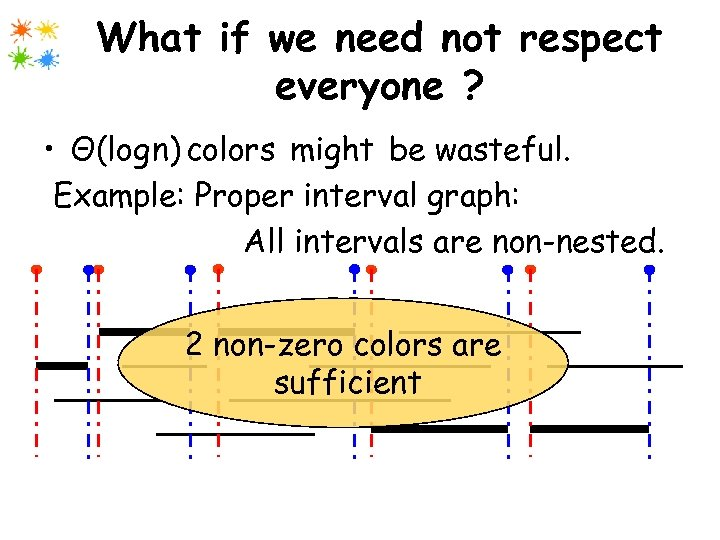What if we need not respect everyone ? • Θ(log n) colors might be wasteful. Example: Proper interval graph: All intervals are non-nested. 2 non-zero colors are sufficient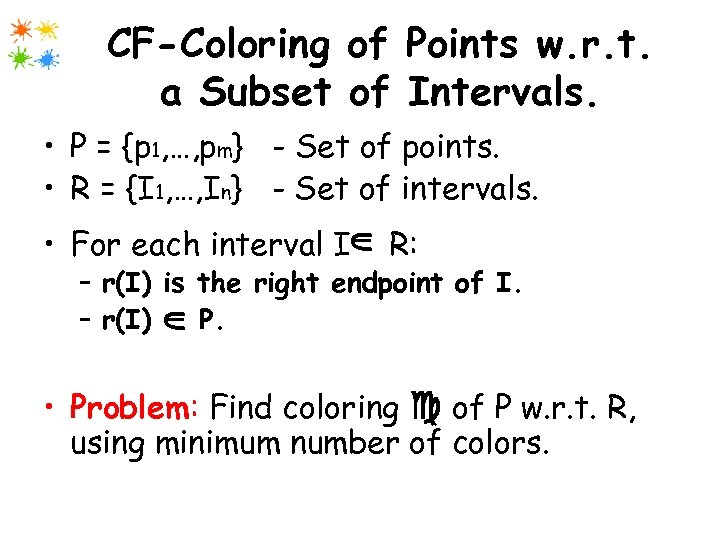CF-Coloring of Points w. r. t. a Subset of Intervals. • P = {p 1, …, pm} - Set of points. • R = {I 1, …, In} - Set of intervals. l U • For each interval I R: l U – r(I) is the right endpoint of I. – r(I) P. • Problem: Find coloring of P w. r. t. R, using minimum number of colors.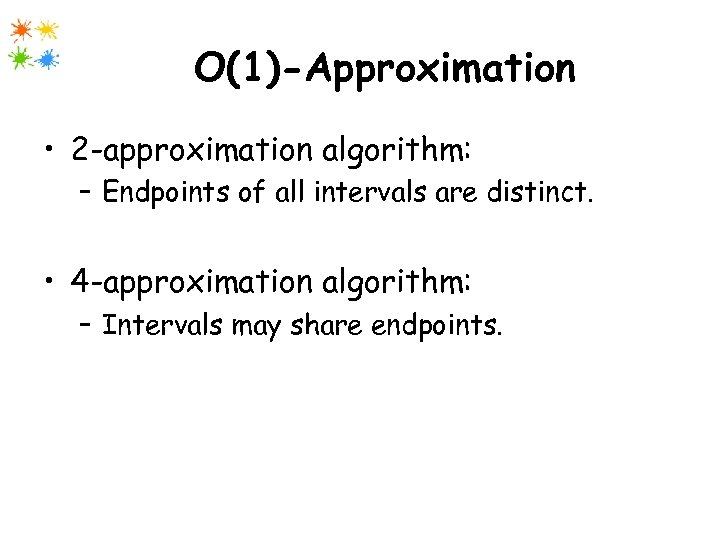O(1)-Approximation • 2 -approximation algorithm: – Endpoints of all intervals are distinct. • 4 -approximation algorithm: – Intervals may share endpoints.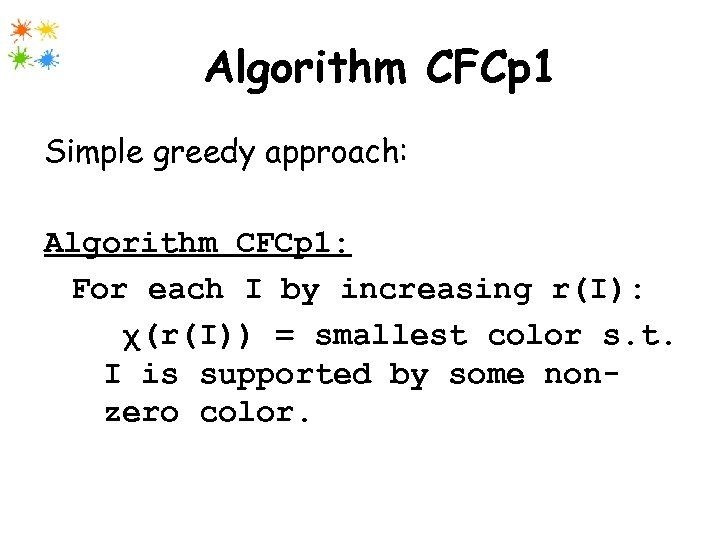Algorithm CFCp 1 Simple greedy approach: Algorithm CFCp 1: For each I by increasing r(I): χ(r(I)) = smallest color s. t. I is supported by some nonzero color.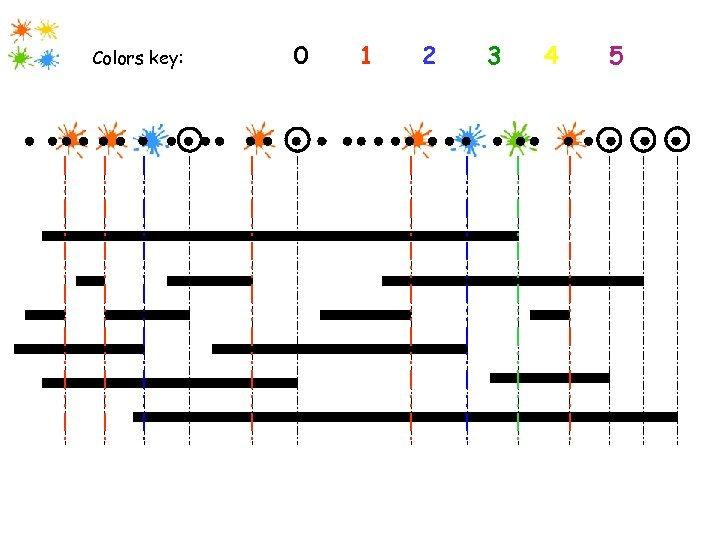Colors key: 0 1 2 3 4 5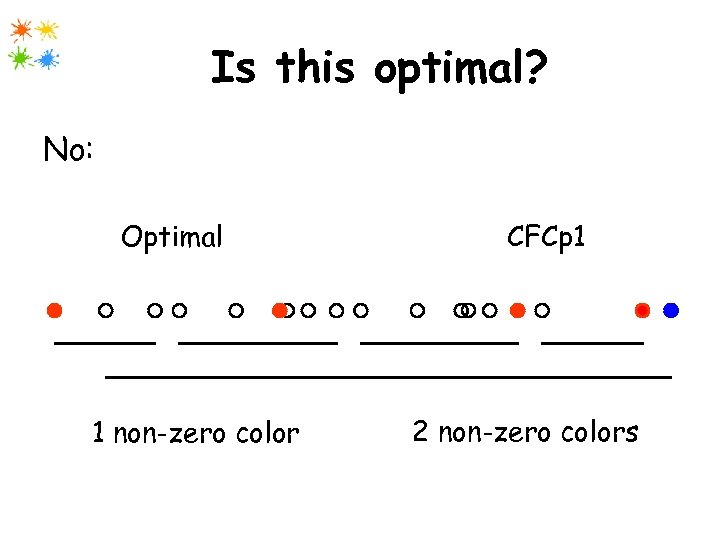Is this optimal? No: Optimal 1 non-zero color CFCp 1 2 non-zero colors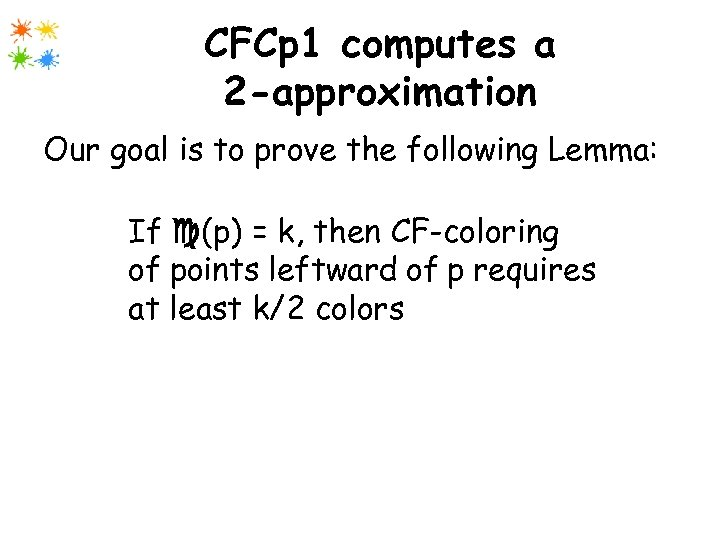CFCp 1 computes a 2 -approximation Our goal is to prove the following Lemma: If (p) = k, then CF-coloring of points leftward of p requires at least k/2 colors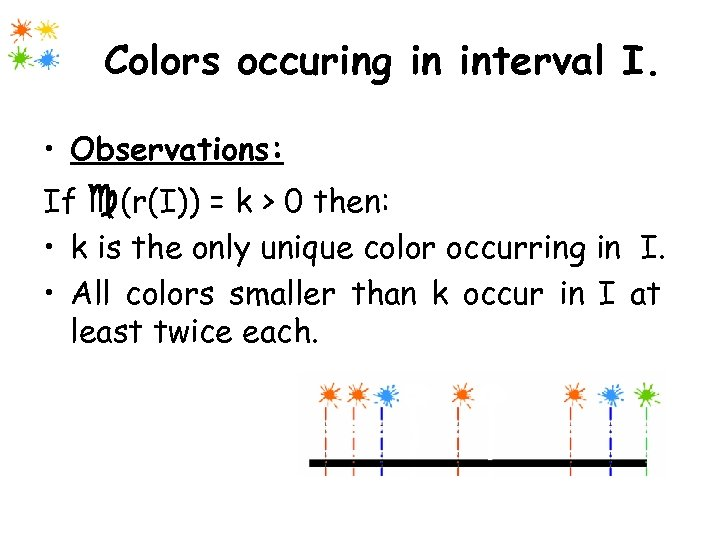Colors occuring in interval I. • Observations: If (r(I)) = k > 0 then: • k is the only unique color occurring in I. • All colors smaller than k occur in I at. least twice each.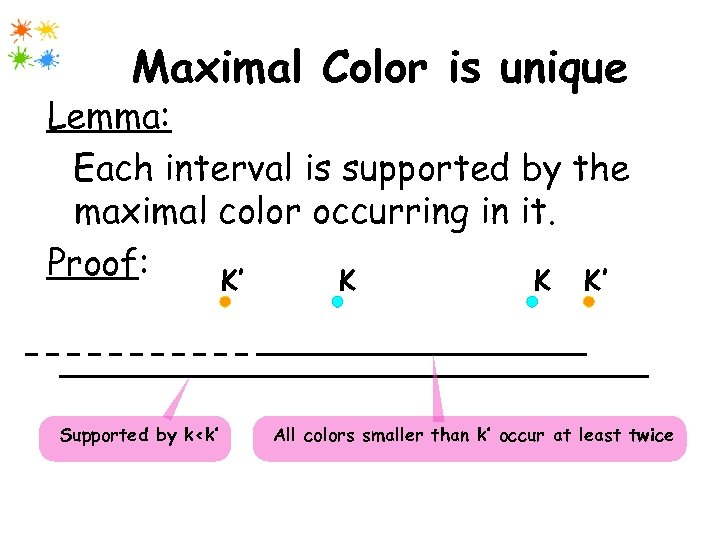Maximal Color is unique Lemma: Each interval is supported by the maximal color occurring in it. Proof: K’ K K K’ Supported by k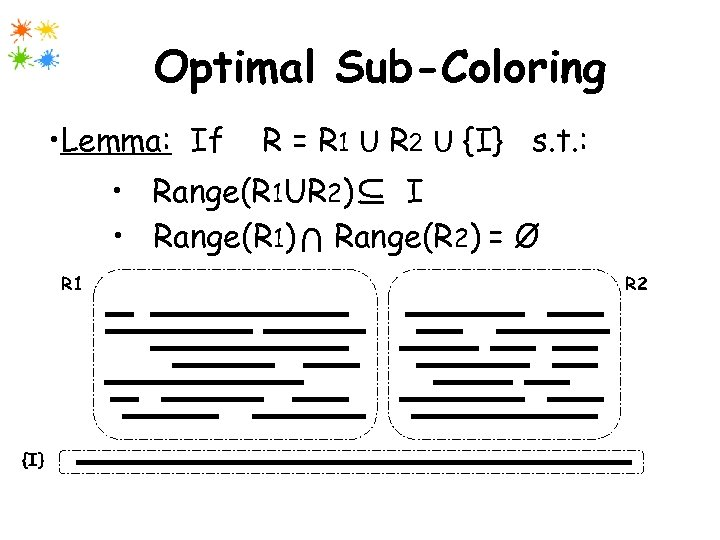Optimal Sub-Coloring • Lemma: If R = R 1 U R 2 U {I} s. t. : Ul • Range(R 1 UR 2) I • Range(R 1) Range(R 2) = Ø U R 1 {I} R 2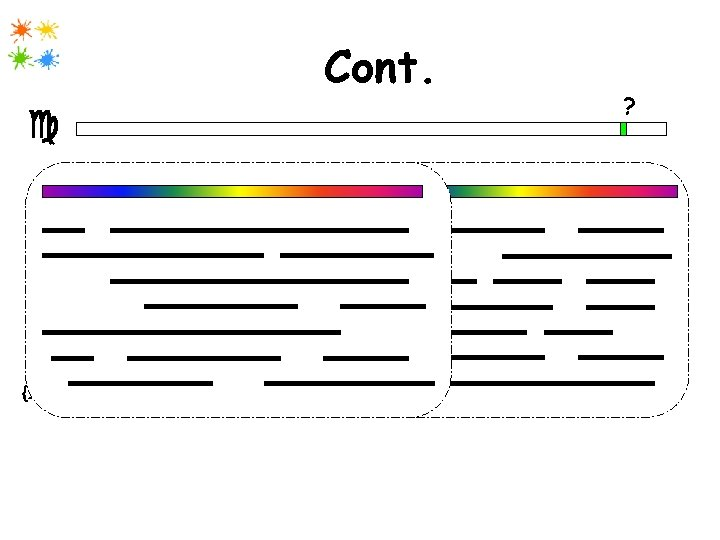Cont. ? R 1 {I} 1 2 R 2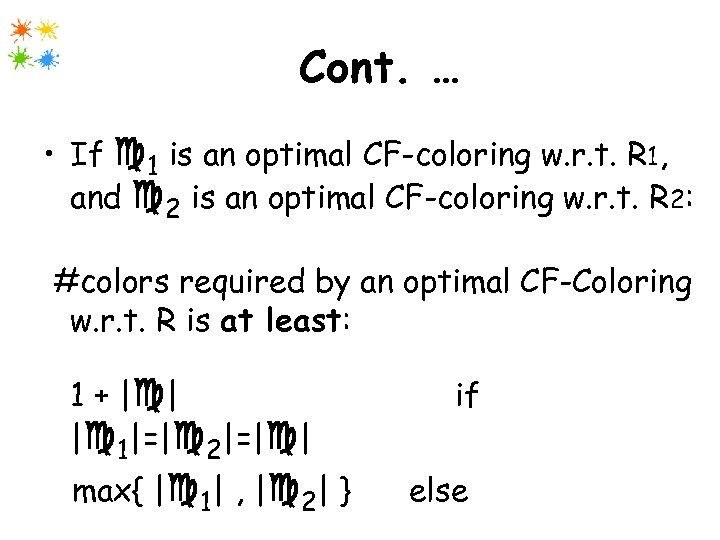Cont. … • If 1 is an optimal CF-coloring w. r. t. R 1, and 2 is an optimal CF-coloring w. r. t. R 2: #colors required by an optimal CF-Coloring w. r. t. R is at least: 1 + | | | 1|=| 2|=| | max{ | 1| , | 2| } if else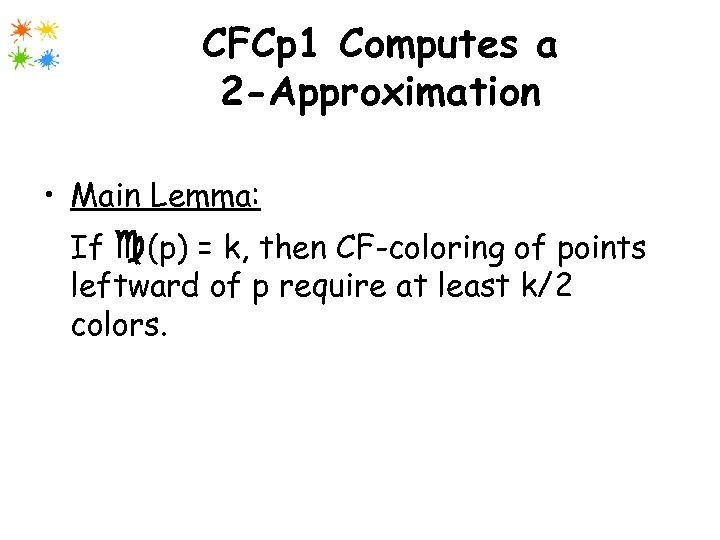CFCp 1 Computes a 2 -Approximation • Main Lemma: If (p) = k, then CF-coloring of points leftward of p require at least k/2 colors.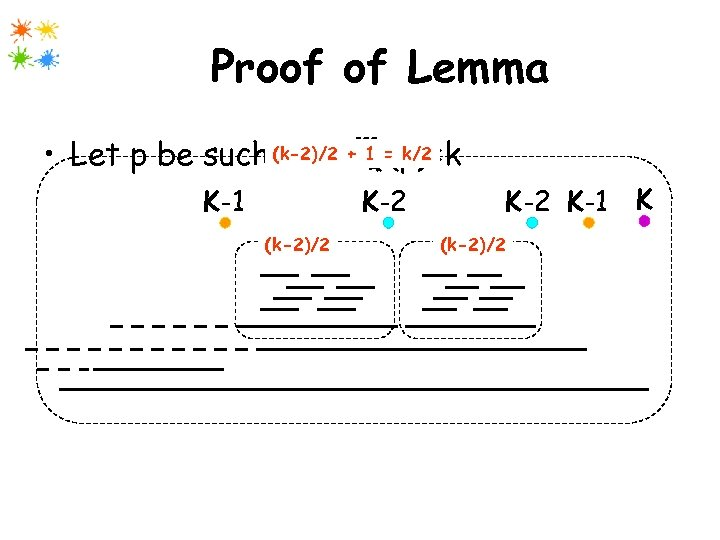Proof of Lemma • Let p be such (k-2)/2 + = k/2 that 1 (p)=k K-2 K-1 (k-2)/2 K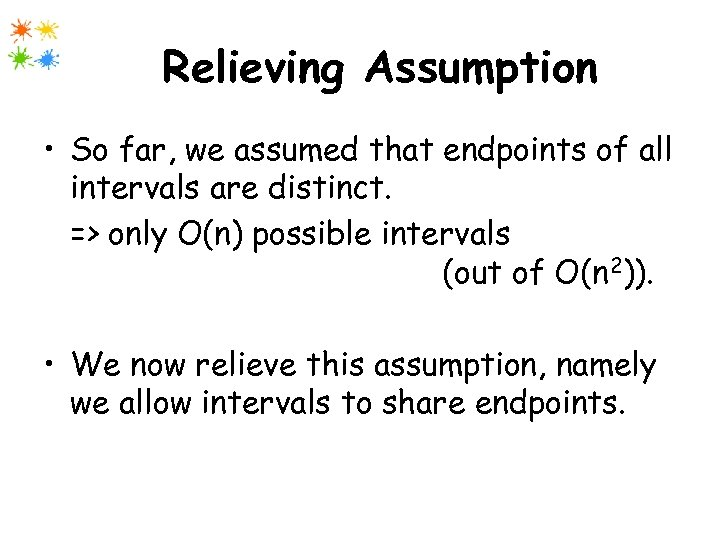Relieving Assumption • So far, we assumed that endpoints of all intervals are distinct. => only O(n) possible intervals (out of O(n 2)). • We now relieve this assumption, namely we allow intervals to share endpoints.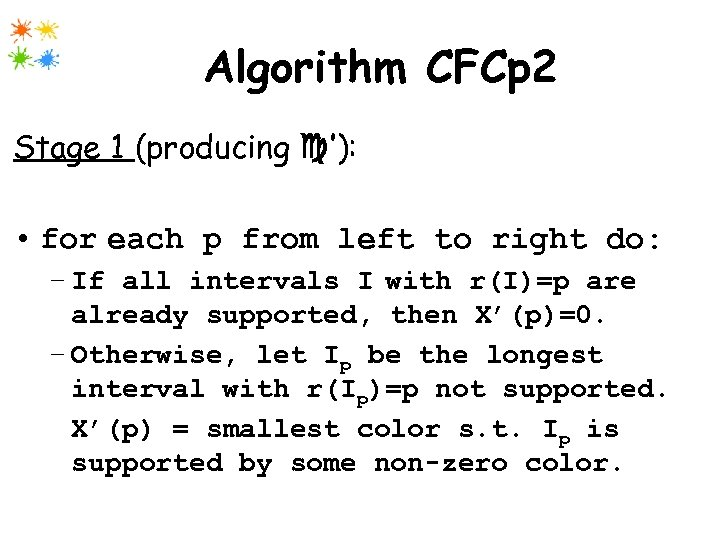Algorithm CFCp 2 Stage 1 (producing ’): • for each p from left to right do: – If all intervals I with r(I)=p are already supported, then Χ’(p)=0. – Otherwise, let Ip be the longest interval with r(Ip)=p not supported. Χ’(p) = smallest color s. t. Ip is supported by some non-zero color.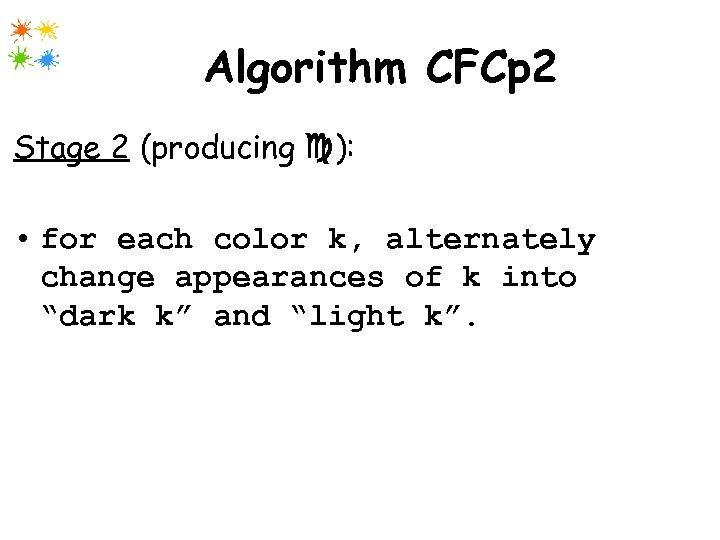Algorithm CFCp 2 Stage 2 (producing ): • for each color k, alternately change appearances of k into “dark k” and “light k”.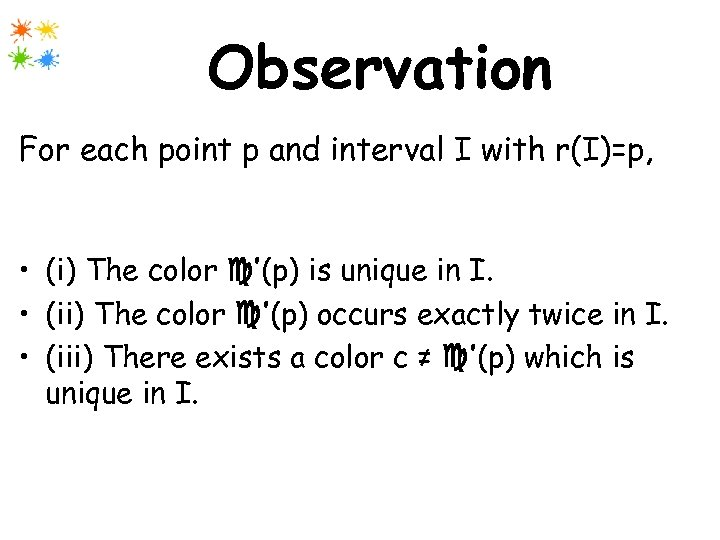Observation For each point p and interval I with r(I)=p, at least one of the following holds at the end of the first stage. • (i) The color ’(p) is unique in I. • (ii) The color ’(p) occurs exactly twice in I. • (iii) There exists a color c ≠ ’(p) which is unique in I.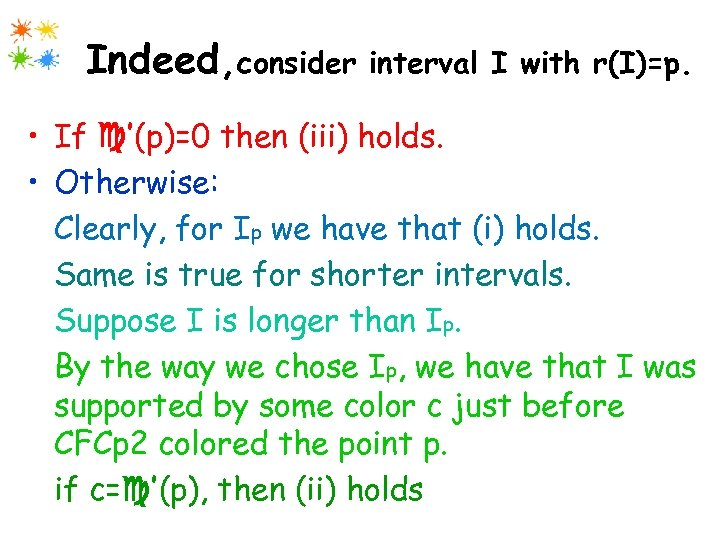Indeed, consider interval I with r(I)=p. • If ’(p)=0 then (iii) holds. • Otherwise: Clearly, for Ip we have that (i) holds. Same is true for shorter intervals. Suppose I is longer than Ip. By the way we chose Ip, we have that I was supported by some color c just before CFCp 2 colored the point p. if c= ’(p), then (ii) holds, otherwise (iii).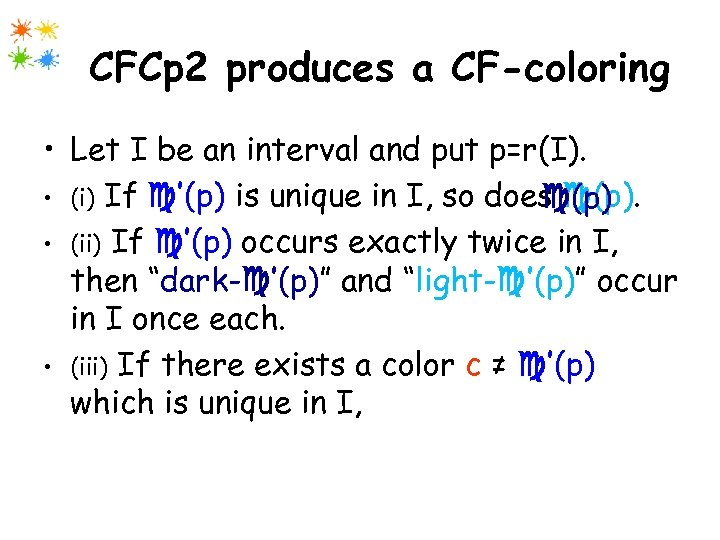CFCp 2 produces a CF-coloring • Let I be an interval and put p=r(I). • (i) If ’(p) is unique in I, so does (p) • (ii) If ’(p) occurs exactly twice in I, then “dark- ’(p)” and “light- ’(p)” occur in I once each. • (iii) If there exists a color c ≠ ’(p) which is unique in I, then either “dark-c” or ”light-c” occur in I exactly once.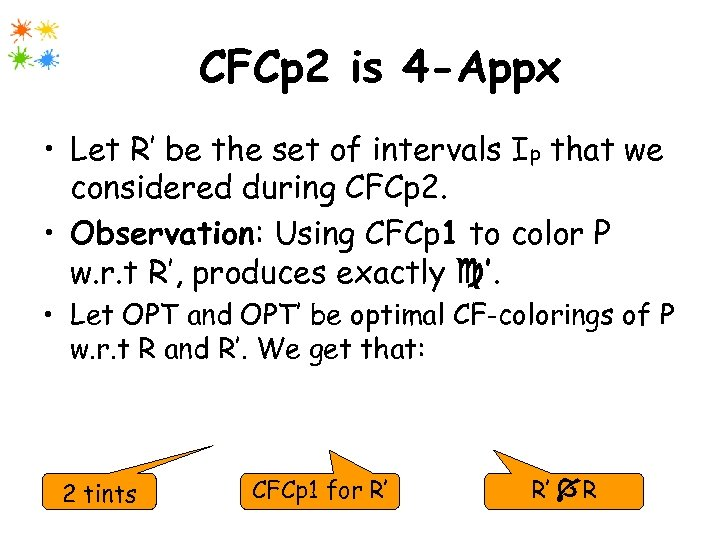CFCp 2 is 4 -Appx • Let R’ be the set of intervals Ip that we considered during CFCp 2. • Observation: Using CFCp 1 to color P w. r. t R’, produces exactly ’. • Let OPT and OPT’ be optimal CF-colorings of P w. r. t R and R’. We get that: | | ≤ 2 | ’| ≤ 4|OPT| 2 tints CFCp 1 for R’ R’ RTHANKS!